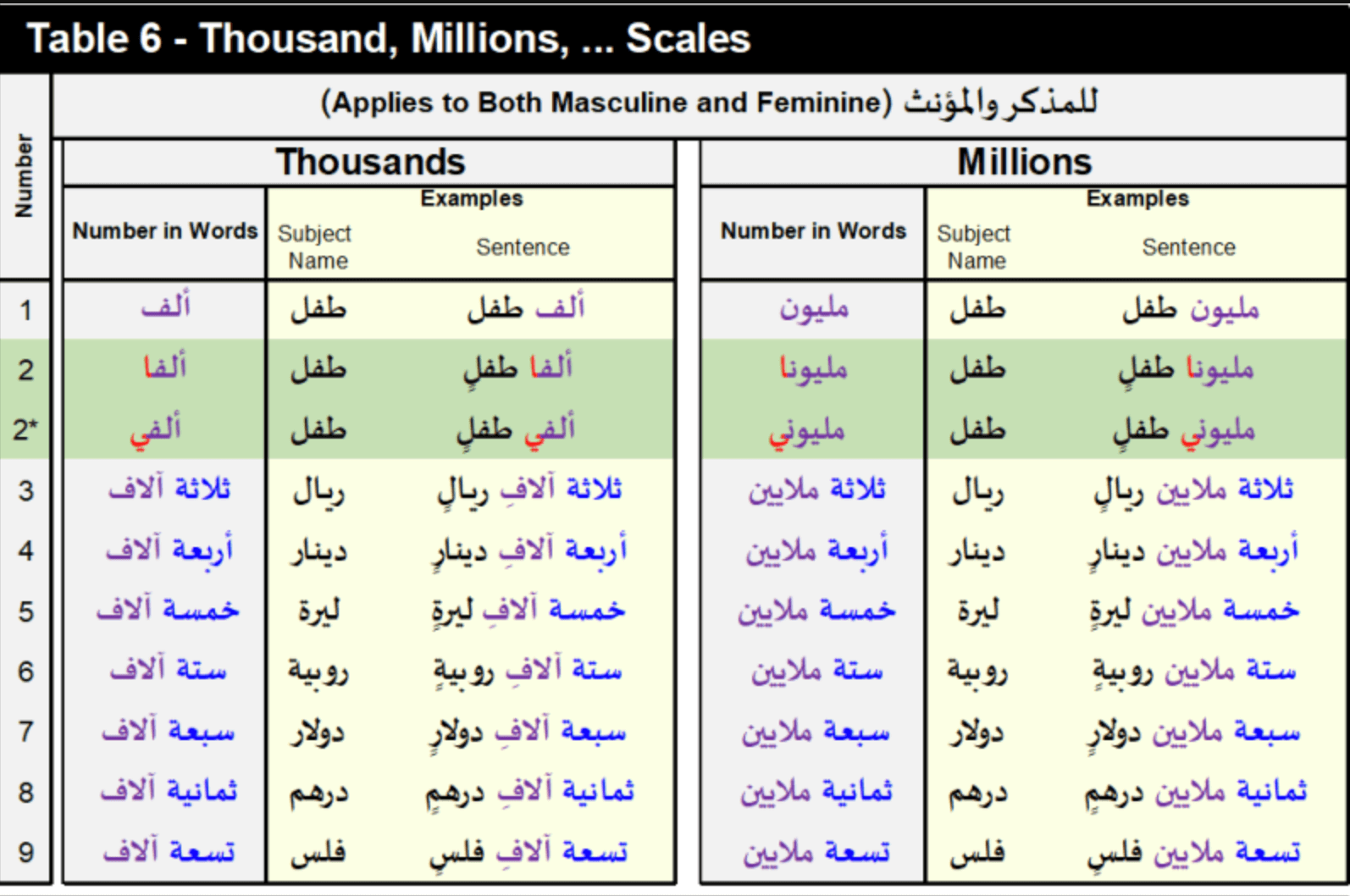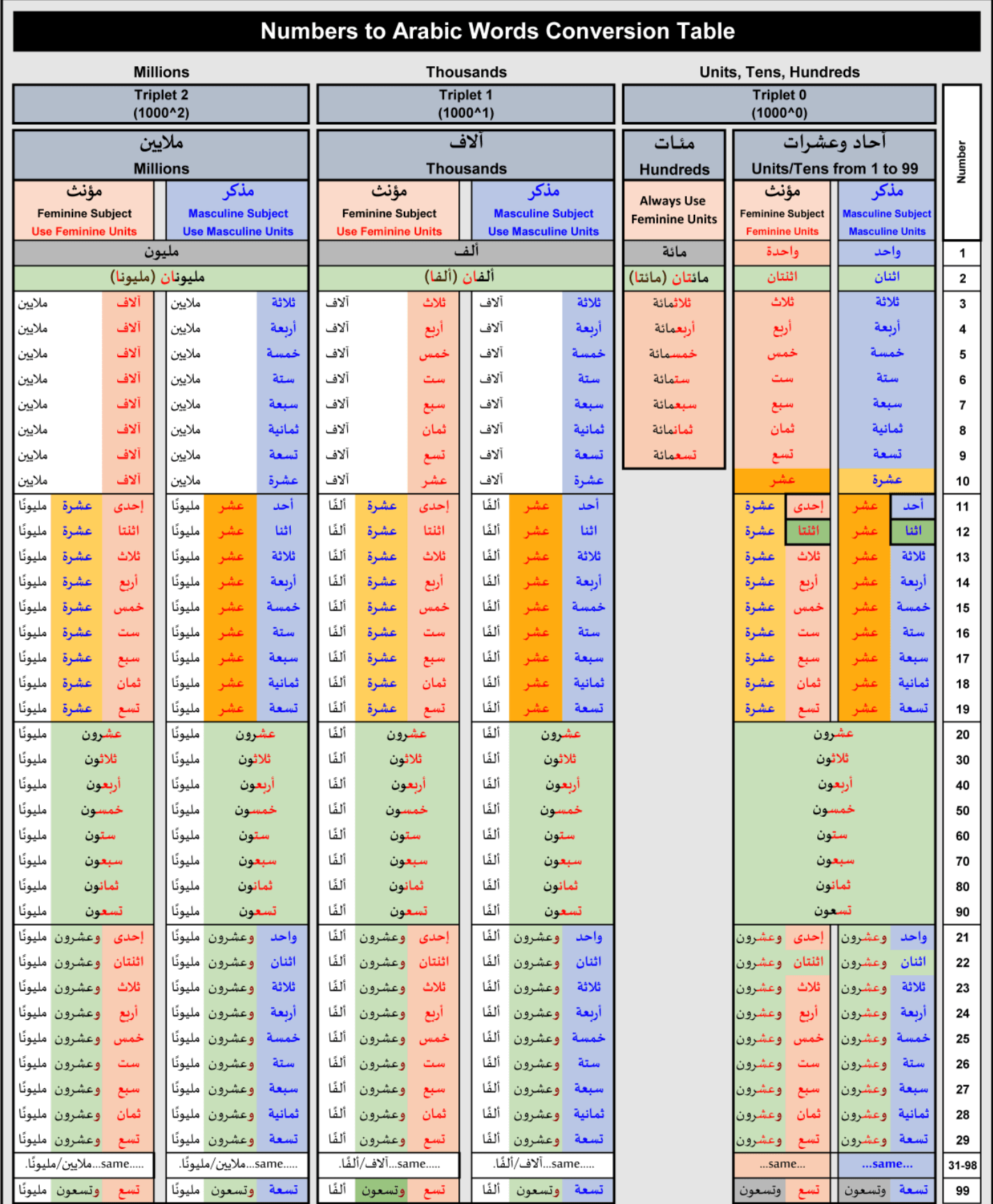# Type the number that you would like to convert to Arabic words!

Produce output text including the subject name
Money

Other Subjects

## What is the Convert numbers to Arabic words tool?

The purpose of creating this tools is to providethe ability to correctly convert numbers to Arabic words based on the following rules:

• Grammatically correct Arabic text for integer numbers from 0 up to 10^21.
• Gender-Sensitive Subjects (Masculine and Feminine (مذكر ومؤنث)).
• Nominative, Accusative, and Genitive Arabic grammar cases (رفع، جر، ونصب).
• Correct positioning of subject names for ones and twos.
• The facility to include the subject name to be counted in the output text; correctly positioned for the appropriate number.
• Address and cover the different forms and standards of writing numbers in words as used in the different Arabic-speaking countries.
• Provides (Advance Settings) as options with the defaults being the most accepted forms of standards.
• Provide the ability to produce output numbers in a legally unambiguous form.
• ## The tools uses the following common grammar rules as default:

• Nominative Grammar Case (حالة الرفع).
• Masculine Subject.
• The Arabic Short Scale Numbering Systems (i.e. Short Scale with Miliard (مليار)).
• The word "مائة" for Hundreds. Note hundred when written as "مائة" must always be pronounced as "مئة".
• Standalone number; i.e. no text is assumed to be added after the resulting output text.
• Maximum scale of Sextillion (سكستليون) i.e. 10^21.
• All of the defaults rules may be changed with the advanced settings options.

## Number to Arabic Words Translation TablesSummary Conversion Rules:

• The above Table is for both the Nominative case and the Accusative/Genitive case, with the execption of the word for the number 2 (as shown).
• For the Numbers 1 and 2, the Subject Name (المعدود) (either Masculine or Feminine) shall be before the Number (العدد) and its tashkеel form depends on the sentence.
• For the Numbers 3 to 10, the Subject Name (المعدود) (either Masculine or Feminine) shall follow the Number (العدد) and shall be in the Plural Form (جمع) and be in "Kasr/Jar" (كسر/جر) form.
•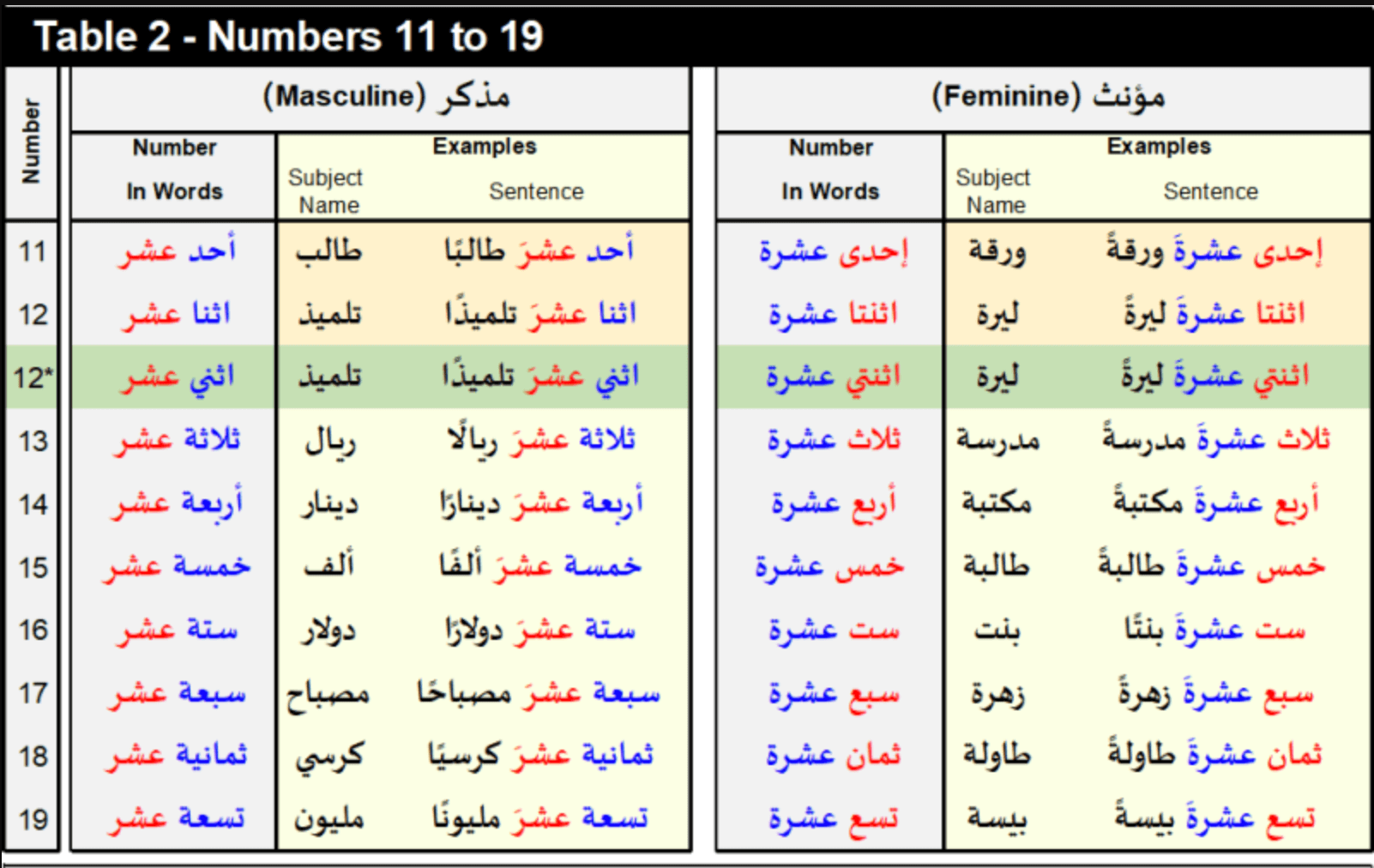Summary Conversion Rules:

• The Unit Part of the Number follows the respective Masculine and Feminine words in accordance with Table-1, except for the Numbers 11 and 12.
• The Masculine word is made of the Masculine Unit Name (1-9) followed by the word "عشرَ".. Except for the numbers 1 and 2, which use "أحد" and "اثنا'" respectively.
• The Feminine word is made of the Feminine Unit Name (1-9) followed by the word "عشرةَ". Except for the numbers 1 and 2, which use "أحد" and "اثنا" respectively.
• The above Table is for BOTH the Nominative and the Accusative/Genitive cases, with the execption of the word for the number 12 (as shown).
• The Subject Name (المعدود) (either Masculine or Feminine) shall follow the Number (العدد) and shall be in the Singular Form (مفرد) and be in the "Tanween Fatih/Nasb" (فتح/نصب) form.
•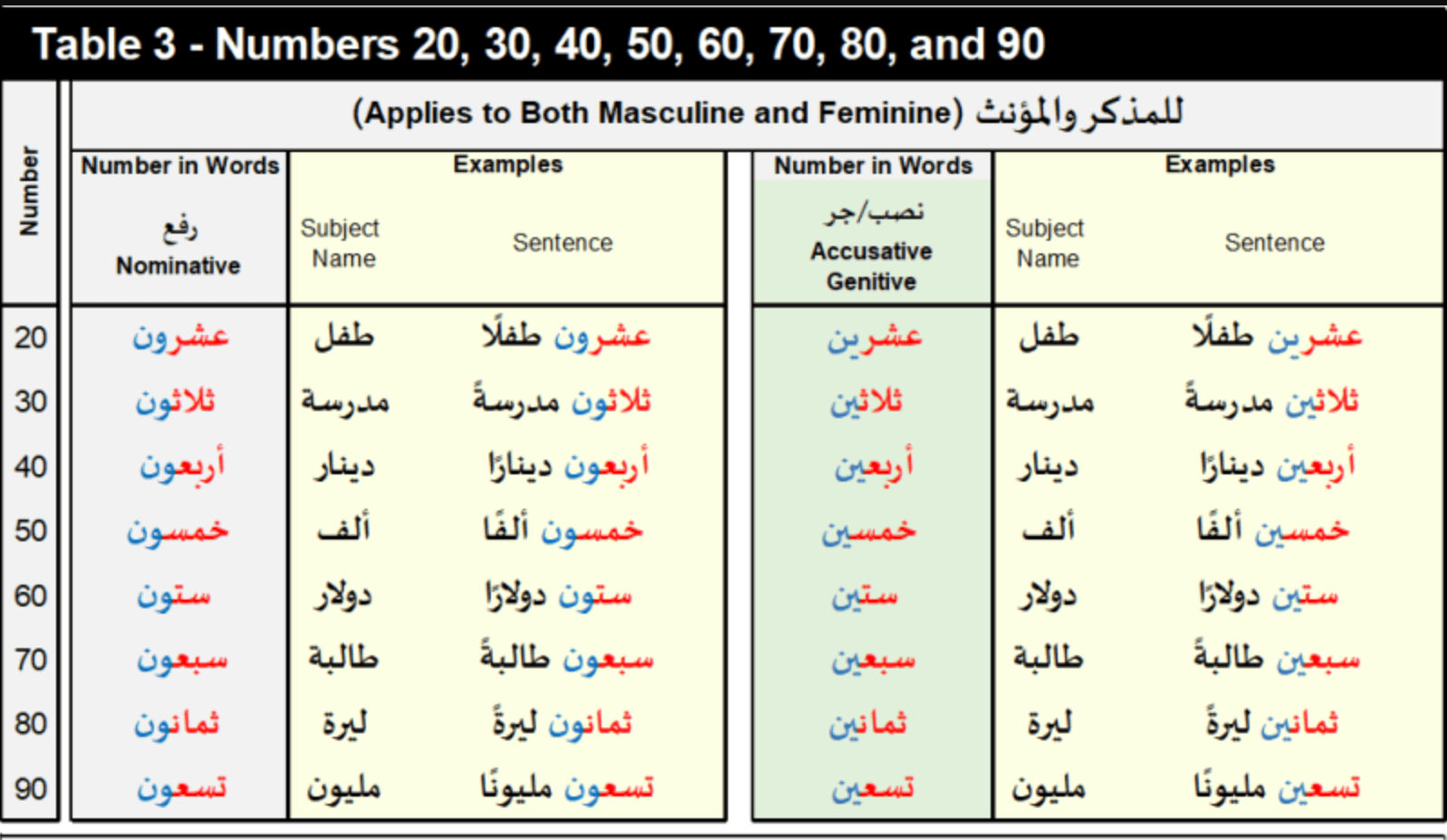Summary Conversion Rules:

• Both the Masculine and Feminine use the Feminine Unit Names (1-9) (see Table-1). with the addition of the letters "ug" in case of nominative case (default) or the letters "" in case of accusative/genitive
• The Subject Name (المعدود) (either Masculine or Feminine) shall follow the Number (العدد) and shall be in the Singular Form (مفرد) and be in "Tanween Fatih/Nasb" (فتح/نصب) form.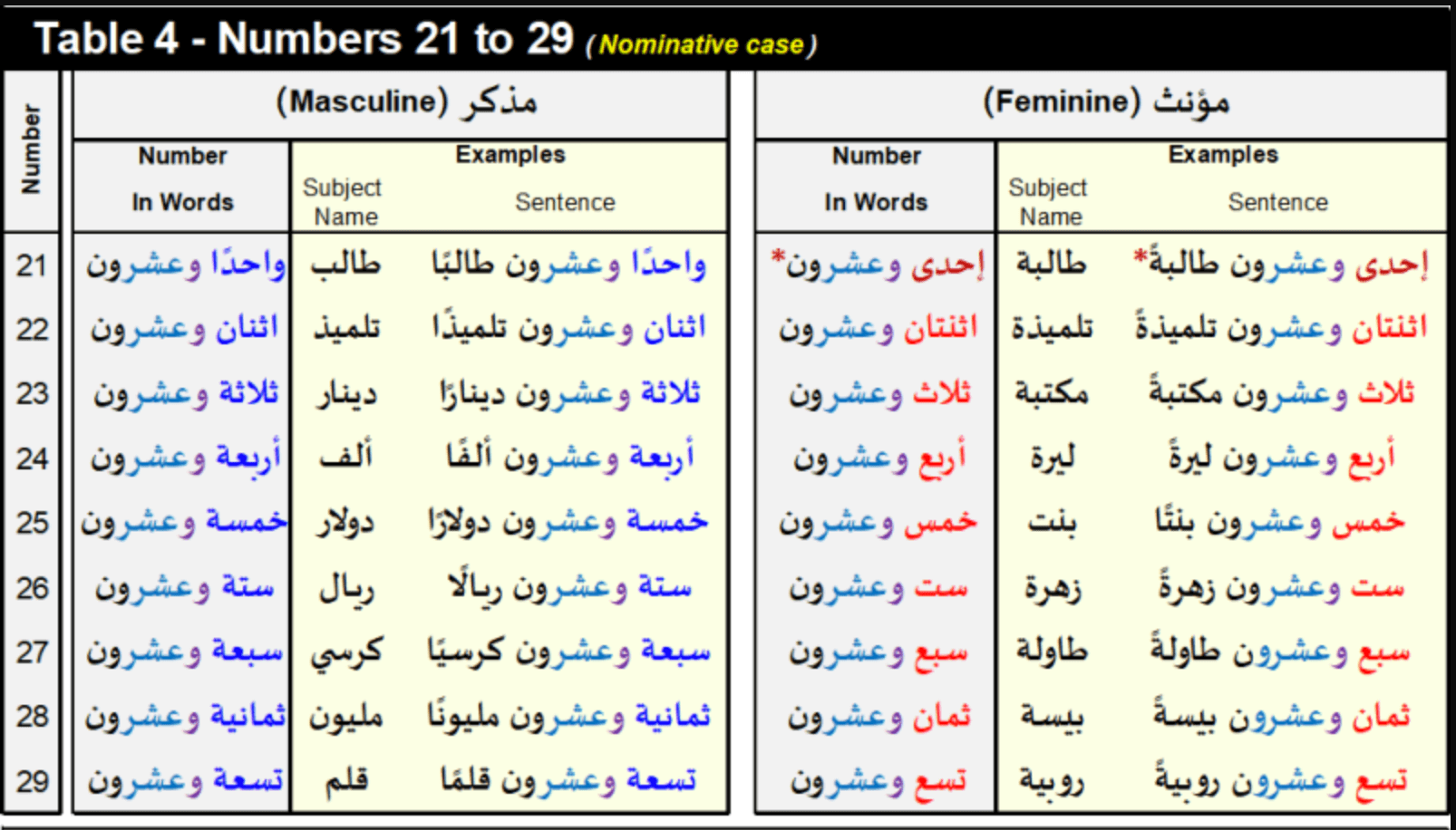Summary Conversion Rules:

• The Unit Part of the Number follows the respective Masculine and Feminine words in accordance with Table-1, except for the Numbers 1 in the Feminine case.
• The word for Number 20 is in accordance with Table-3.
• The letter "و" (i.e. АND) is added before the word for 20 (without spaces).
• The Subject Name receives "Tanween Fatih".
• The above Table is for the Nominative case. See Table-3 for the Accusative/Genitive case (i.e. the ".("وعشرين" :shall be replaced with the word "وعشرون" :word
• The words for the Numbers 31 to 99 follow the same logic using the above Table and Table-3.
• The Subject Name (المعدود) (either Masculine or Feminine) shall follow the Number (العدد) and shall be in the Singular Form (مفرد) and be in "Tanween Fatih/Nasb" (فتح/نصب) form.
•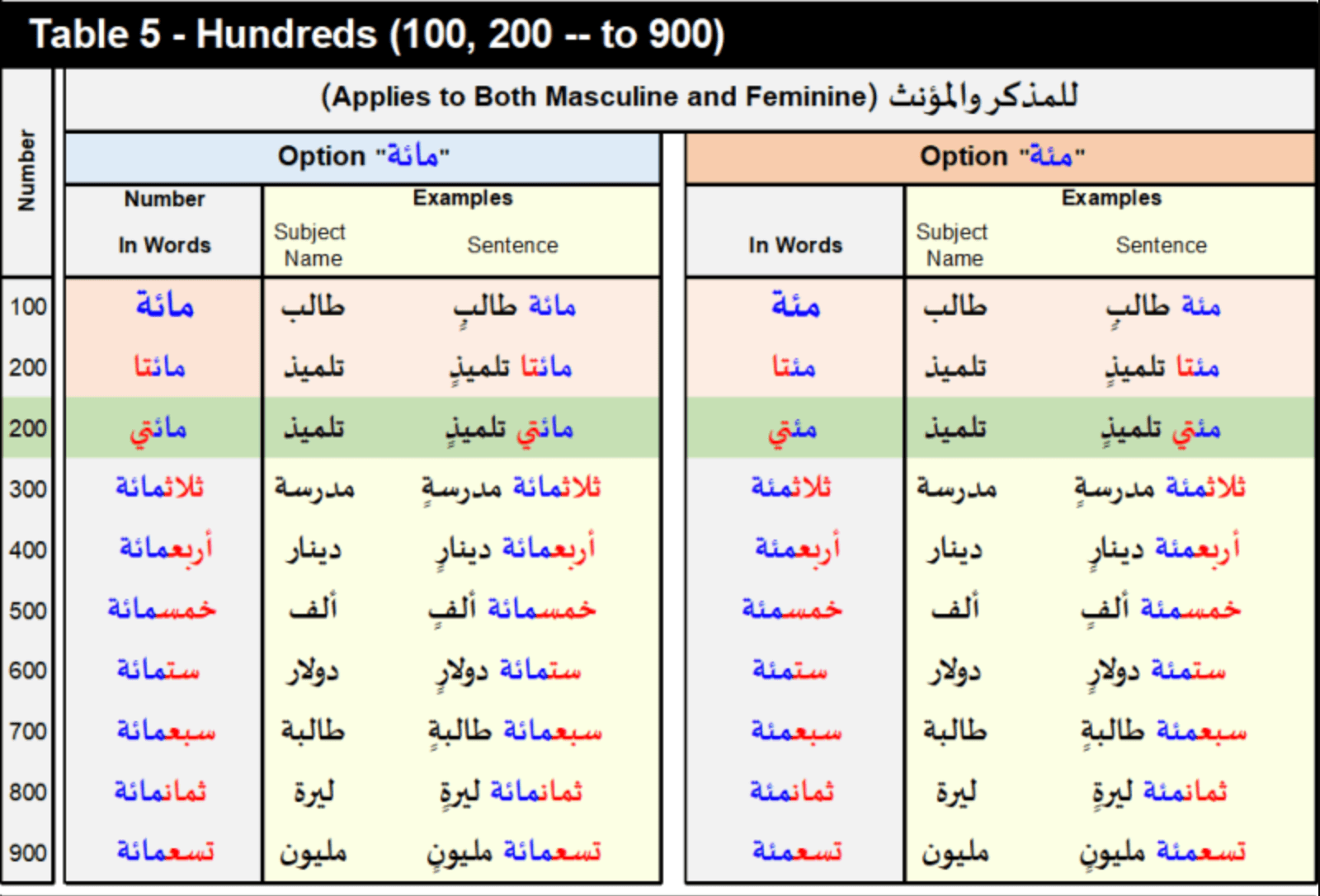Summary Conversion Rules:

• Both the Masculine and Feminine use the Feminine Unit Names (1-9) (see Table-1), except for the Numbers 1 and 2 which shall be as shown above.
• The Subject Name (المعدود) (either Masculine or Feminine shall follow the Number (العدد) and shall be in the Singular Form (مفرد) and be in "Tanween Kasr/Jar" (كسر/جر) form.